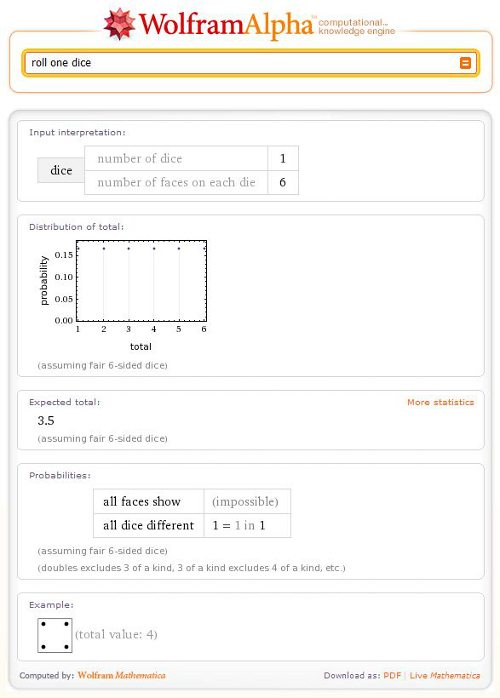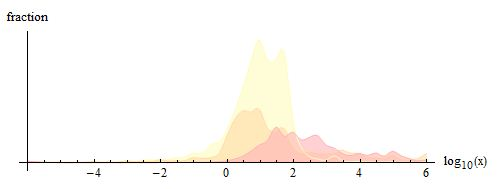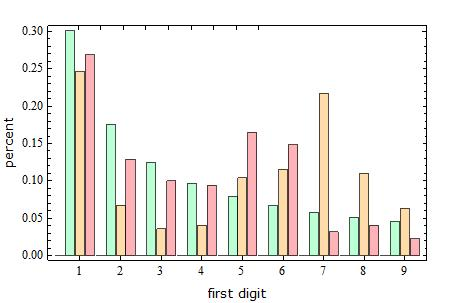# The Curious Case of Benford’s Law

December 13, 2010 —Comments Off

When you roll dice, all numbers have the same probability to show up (assuming that the dice aren’t loaded in any way):However, the leading digits of numbers in very large accumulated datasets—for example, the amount you pay for each household bill over the course of a year—follow a very different pattern. In such cases it is much more likely that a given number will start with one, with decreasing probability for each higher digit up to nine. This statistical phenomenon is called Benford’s law.

Benford’s law arises naturally if the data under consideration span several orders of magnitude—for example, the first digits of the powers of two obey Benford’s law:This plot shows the frequency of initial digits for every number from 2^1 to 2^1000:Benford’s law seems to apply to a broad variety of datasets, not just pure mathematical progressions, and it has a number of serious applications. It is often used to detect anomalies in datasets, including income taxes; most people don’t know about Benford’s Law, so when they fill out fraudulent tax forms, they tend to choose numbers with higher leading digits. If the distribution of leading digits in a given return doesn’t closely follow Benford’s predicted distribution, that could be a sign that the return should be pulled for additional review.

Wolfram|Alpha’s mission is to make the world’s knowledge computable, and we already have trillions of bits of data covering hundreds of different domains—ideal conditions to test Benford’s law. Consider the distribution of leading digits for values of physical quantities. When we plot the digits for all values in Wolfram|Alpha user queries with units of inches, seconds, and British pounds, each of them closely follows Benford’s law.(Probabilities of the first digits according to Benford’ s law (green) and for inches (yellow), seconds (brown), and British pound (red), respectively.)

To observe the validity of Benford’s law for a dataset, the scale of the data must extend over several orders of magnitude. If we plot the magnitude of the numerical prefactors of all the values above, the data clearly span several orders of magnitude.Not all datasets follow Benford’s law, of course. Also, see below the first-digit probabilities for Wolfram|Alpha user inputs in units of kilograms and feet. Here, the distinctive variation from Benford’s law has anthropological reasons: the average height and weight of humans are in the 5–6 feet and 70–80 kg ranges, respectively (see one of our recent blog posts).(Probabilities of the first digits according to Benford’s law (green) and for kilograms (brown) and feet (red).)

Benford’s law is indeed a curious little law.

This post was written by Michael Trott and Bjorn Zimmermann.

What you’ve omitted is what emerges from a quick thought experiment: what if aliens evolved on a planet with 8 or 12 fingers and had similar mathematical skills to ours? Would there be a Benford’s Law for them too?

Obviously, yes. It’s a law which applies across number bases.

Which means that this could be appled to all sorts of other non-base10 versions of the same datasets. So octal dumps or hex dumps of data where you don’t actually know anything more than that it’s meant to represent a dataset.

Posted by Charles Arthur December 14, 2010 at 5:58 am

This statement: “This plot shows the frequency of initial digits for every number from 2^1 to 2^1000”, is incorrect;

It gives the frequency of the initial digits of all of the *powers of two* between 2^1 and 2^1000, hence the numbers totalling 1000. If you wanted to do this for all of the numbers between 2^1 and 2^1000, you would have to wait an exceptionally long time, while it works out the first integer digit of 10715086071862673209484250490600018105614048117055336074437503883703510511249361224931983788156958581275946729175531468251871452856923140435984577574698574803934567774824230985421074605062371141877954182153046474983581941267398767559165543946077062914571196477686542167660429831652624386837205668069376 numbers, which are evenly distributed.

Posted by Will Brigg November 4, 2014 at 10:20 am